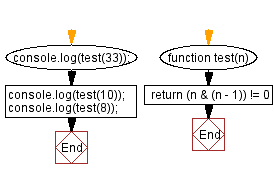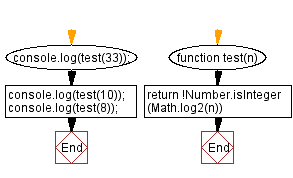# JavaScript: Check sum of consecutive positive integers

## JavaScript Math: Exercise-73 with Solution

Write a JavaScript program to check if a given positive integer can be expressed as the sum of two or more consecutive positive integers.

Sample Data:
33 can be represented as 11 + 22
10 = 1+2+3+4
but 8 cannot be represented in this way.

Sample Solution-1:

HTML Code:

``````<!DOCTYPE html>
<html>
<meta charset="utf-8">
<title>JavaScript program to check sum of consecutive positive integers</title>
<body>

</body>
</html>
```
```

JavaScript Code:

``````function test(n)
{
return (n & (n - 1)) != 0
}
console.log(test(33));
console.log(test(10));
console.log(test(8));
```
```

Sample Output:

```true
true
false
```

Flowchart:Live Demo:

See the Pen javascript-math-exercise-73-1 by w3resource (@w3resource) on CodePen.

Sample Solution-2:

JavaScript Code:

``````function test(n){
return !Number.isInteger(Math.log2(n))
}
console.log(test(33));
console.log(test(10));
console.log(test(8));
```
```

Sample Output:

```true
true
false
```

Flowchart:Live Demo:

See the Pen javascript-math-exercise-73-2 by w3resource (@w3resource) on CodePen.

Improve this sample solution and post your code through Disqus

What is the difficulty level of this exercise?

Test your Programming skills with w3resource's quiz.

﻿